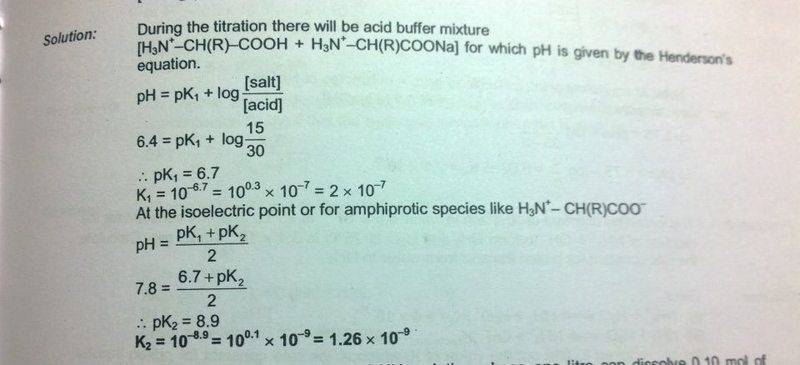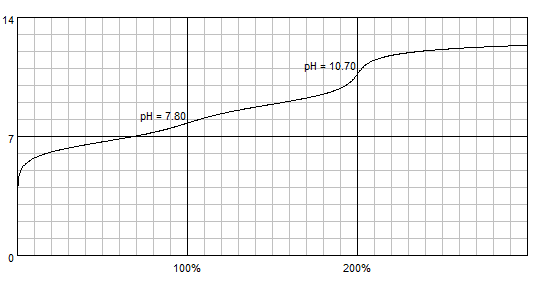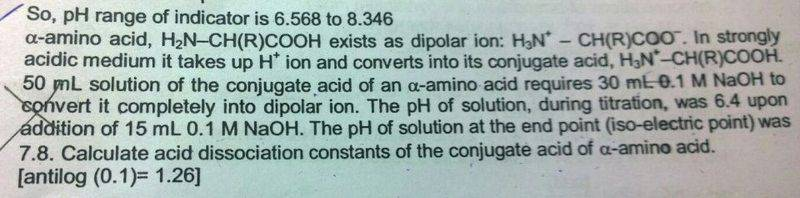# Titration of amino acid with strong base

Gold Member

## Homework Statement

An amino acid H2N-CHR-COOH e its as a dipolar ion:
+H3N-CHR-COO-. In strongly acidic medium, it takes H+ to form conjugate acid
+H3N-CHR-COOH
50ml solution of conjugate acid required 30ml of 0.1M NaOH to completely convert into dipolar ion. The pH of solution during titration was6.4 upon addition of 15ml of 0.1M NaOH. The pH of the solution at the end point was 7.8. Calculate acid dissociation constants of the conjugate acid of amino acid.

None

## The Attempt at a Solution

The solution contains conjugate acid which is in equilibrium.
H2N-CHR-COOH↔H2N-CHR-COO-+H+
During titration, buffer is formed.
When NaOH is added,
H2N-CHR-COOH+OH-→H2N-CHR-COO-+H2O
Hence change in pH is resisted.
The concentration of H2N-CHR-COO- from conjugate acid is negligible. Its equivalents is 15*0.1. But wouldn't the equivalents of the conjugate acid change since its reacting with NaOH? But my teacher told me that its constant. Why should it be constant?
What I mean is, initially there was 3 milli moles of conj. acid.
After adding 1.5 milli moles NaOH, 1.5 milli moles of dipolar ion is formed and 1.5 milli moles of acid are left. Hence ##\frac{salt}{acid}=0## after reaction and ##pH=pk_a##.
But he said its ##pH=pk_a+log\frac{15}{30}##
In Atkins chemical principles, he discusses titration with triprotic acid H3A2+. At one point all the acid converts to H2A+. That means the concentration does change.

Last edited:

## Answers and Replies

Gold Member
@Borek , Can you please help me with this problem? Anyone?
This is the solution given.Just explain the buffer part for me. I was able to solve the rest myself.
Why is [acid] 30 even after reaction with NaOH?

Borek
Mentor
The solution given is definitely wrong. This is how the titration curve for a substance with pKa1 = 6.7 and pKa2 = 8.9 looks like:(calculated with BATE). End point is 10.7, not 7.8.

Solution - as given - doesn't make much sense. You are right about the 15/30 part - it is simply wrong. Then, isoelectric point is not the endpoint, so the equation for isoelectric point doesn't apply.

•Titan97
Gold Member
Thank you @Borek.
I have edited the question. There is a printing mistake. (I had to refer my friend's book)
Edit "pH of solution at end-point (or isoelectric point) was 7.8"
I my book, the letters inside the bracket was faded.
But why should the end point be I so electric point?
So this means that the total concentration of acid has changed since the amount of NaOH added is not negligible. Also, pH=pKa1.
Am I correct?

Last edited:
Borek
Mentor
Neither question nor the solution makes sense to me.

And no, if you need 30 mL of base to completely neutralize the acid, after 15 mL you are not at the pH=pKa1 point. You are at the first equivalence point, that's not the same.

Gold Member
If 30 ml of 0.1M NaOH is required for complete neutralisation, then there are 3 millimoles of acid initially. 1.5 milli moles of NaOH on reacting with acid gives 1.5 milli moles of salt. Hence remaining acid is 1.5 milli moles.
[acid]=1.5/65
[salt]=1.5/65
(Total volume of solution after adding NaOH is 15+50)
So buffer will have pH=pKa1

Borek
Mentor
No, this is a diprotic acid, you are again lousy about what is the acid and what is the conjugate base.

Gold Member
The reaction is ##^+NH_3-CHR-COOH+NaOH\rightarrow ^+NH_3-CHR-COONa+H_2O##
Initial milli moles of acid is 3.
1 mole of acid on reaction with NaOH gives 1 mole of salt. So 1.5 milli moles of salt will be formed when pH=6.4
Remaining acid is 1.5 milli moles.

Borek
Mentor
1 mole of acid on reaction with NaOH gives 1 mole of salt.

You are aware of the fact this salt of yours is another acid? You are stubbornly ignoring the fact you have TWO acid/conjugate pairs, and each one is a separate buffer.

Besides, you will first lose proton from the -NH3+, not from -COOH.

•Titan97
epenguin
Homework Helper
Gold Member
It is too hard to help you because hard to understand what the question or givens really are. We could probably help if you reproduced the question photographically like you have the solution. I have at least one problem with this solution: you talk of dipoolar ions but there is given a thing represented as +NH3CHRCOONa. That is not a dipoolar ion and it is nothing that really exists, the dipolar ion you wrote correctly in your first sentence of #1.

I really dont know what you mean by 'end point', if thisn is still in the question please explain.

•Titan97
Gold Memberepenguin
Homework Helper
Gold Member
My best guess is this is a somewhat trick question and the amino acid is histidine which has three ionizable groups and the IEP would be between pKa2 and pKa3, and in fact near 7.8.

•Titan97
Borek
Mentor
My best guess is that the question and the answer provided are simply wrong•Titan97
epenguin
Homework Helper
Gold Member
My best guess is that the question and the answer provided are simply wrongI agree. I have twice written out an articulated explanation of how to think about this problem, and then lost it! Oof!However straight thinking does not fit the data given, (never mind the redundant). It is not the student's fault. I dread to think this was in a State or University exam. Can we be told where it is from?

•Titan97
Gold Member
This question is taken from an IITJEE coaching institute's textbook. IITJEE is an entrance exam to get admission to Indian institute of Technology which is the best Engineering college in India and one of the top colleges in Asia.
Many students from IIT are successful. One such person is the present CEO of google. He was from IIT.
I am using these books only for practice. Some of the question in IITJEE preparation books don't make sense.

IITJEE is a national level exam for which about 15 lakh students all over India prepare. The questions in the exam are good. But some questions given by coaching centres are of low quality.
Physics and Maths questions from such books are really good. It's just the chemistry part that bothers me.)

For theory, I am referring Atkins and claydon so I can become strong in concepts.

Last edited:
epenguin
Homework Helper
Gold Member
Well yes, we now recognise this coaching Institute's style here, at least the last year or two I have particularly. Use those last books or other standard British of American ones with excercises, or get past exam papers of the IITEE which I hope these are not.I hope useful to illustrate straight thinking, at least my thinking.

What is called 'the conjugate acid' here is +NH3CHRCOOH . You can buy amino acids as such, but also as sodium salts or as hydrochlorides, powders or crystals. This 'conjugate acid' is what you'd get if you dissolved e.g. glycine hydrochloride. Or if to glycine you added an equal number of moles of HCl, as stated (or implied). Plus in each case a molarity of Cl- equal to that of glycine.

Starting with that if you added an equal molarity of NaOH you'd deprotonate this and get practically all into the dipolar form +NH3CHRCOO- for any amino acid with neutral side chains. The pH would be around 6, halfway between pKs. (6.4 is a bit high, but maybe with high salt or strange solvent or nonnatural amino acid...) So if the 15 ml is 1 mole NaOH/mole aa that's approximately OK. But then another 15 ml, another mole/mole aa you'd deprotonate all the -NH3+ and be at very high pH, not 7.8. And there's no way the 1 mole/mole situation wouldn't be the IEP.
If on the other hand 30 ml is one, not two, moles NaOH/mole aa, then 15 ml is 1/2 mol/mol and the pH would be the pKa1, about 2.2.

So maybe this was about an amino acid with another dissociating group? Histidine is the only halfway reasonable one.
Making the solution dissolving histidine dihydrochloride or bringing histidine hydrochloride or histidine there with acid, you'd have +NH3CHR+COOH . Adding 2 mol NaOH/mol his you'd have nearly everything as +NH3CHRCOO- and the pH would be (halfway between imidazol and amino group pKs) around a 7.8 IEP. But then half the base, 1 mol/mol NaOH/his would give you mean of imidazol and carboxylate pKs, about pH 4. Also from two differences between pKs you cannot calculate three, or any, pKs.

So there are rational and efficient ways to think about questions like this, but this question does not stack up.

Last edited:
•Titan97
Gold Member
It was not a previous year question.